# Inport

Convert Simulink input signal to RF Blockset signal

• Library:
• RF Blockset / Circuit Envelope / Utilities

•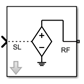## Description

The Inport block imports Simulink® signals into the RF Blockset™ circuit envelope simulation environment. For an introduction to RF simulation, see the example, Simulate High Frequency Components.

Complex-valued input signals Ik(t) + j · Qk(t) are the modulations at the frequencies {fk} specified in the Carrier frequencies parameter of the block.

The input port converts the complex simulink input signals into an RF signal suitable for multicarrier simulation:

$out=\mathrm{Re}al\left(\sum _{k=1}^{N}\left({I}_{k}\left(t\right)+j·{Q}_{k}\left(t\right)\right)·{e}^{j2\pi {f}_{k}t}\right)$

The Source type parameter specifies the Simulink signal as either current, or voltage, or power source. For more information, see How to Use Inport Block.

Inport block mask icons are dynamic and show the current type of source. This table shows you how the icons on this block vary based on the type of source you set on the Source type parameter on the block.

Source type: `Ideal voltage`Source type: `Ideal current`Source type: ` Power`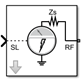## Parameters

expand all

Inport block interpretation of Simulink signal, specified as:

• `Ideal voltage` — The block outputs Simulink signals as voltage signals v(t) in the RF Blockset environment. When you choose an ideal voltage input port you need to manually add a series of source impedance to match the blocks connected to the input port. The following figure illustrates the internal configuration of the block.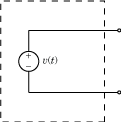• `Ideal current` — The block outputs Simulink signals as current signals i(t) in the RF Blockset environment. When you use an ideal current input port, you manually add a parallel source impedance to match the blocks connected to the input port. The following figure illustrates the internal configuration of the block.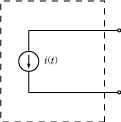• `Power` — The block interprets the Simulink signals, Pv(t), as available power and internally uses a voltage source, and series impedance. You use this option to import a 50 ohm environment or different reference impedance signals created using Communications Toolbox™. When you select this option, the input port automatically inserts a source impedance in your circuit as shown in the following figure.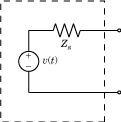The voltage v(t) is a scaling of the Simulink signal vSL(t):

`$v\left(t\right)=2\sqrt{\text{Re}\left({Z}_{s}\right)}{v}_{SL}\left(t\right)$`

In the preceding equation, Zs is the value of the Source impedance (ohms) parameter.

The generator delivers real power to the load Zl:

`${P}_{load}={|v\left(t\right)|}^{2}\frac{\mathrm{Re}\left({Z}_{s}\right)}{|{Z}_{s}+{Z}_{l}|{\text{​}}^{2}}$`

When Zl = Zs*, this generator delivers the available power |vSL(t)|2.

Source impedance for available power match, specified as a vector of positive integers in ohms

#### Dependencies

To enable this parameter, select `Power` in Source type.

Carrier frequencies, specified as a vector of positive integers in Hz. In carrier frequencies, the elements are a combination of fundamental tones and corresponding harmonics in the Configuration block.

Ground RF circuit terminals, specified as a `on` or `off`. Select this parameter to ground and hide the negative terminals. Clear the parameter to expose the negative terminals. By exposing these terminals, you can connect them to other parts of your model.

expand all

expand all

## Version History

Introduced in R2010b

expand all

Behavior changed in R2021b